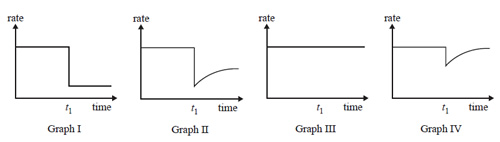Rate of reactions 2010 VCE 1) The factors which influence the rate of reaction between dilute hydrochloric acid and powdered calcium carbonate were investigated. Which one of the following changes would not increase the rate of the reaction? A. stirring the mixture B. heating the reaction mixture C. increasing the concentration of the acid D. replacing the powder with a lump of calcium carbonate Solution2) Reactants A and B are placed in a sealed container with a suitable catalyst where they react according to the equation A(g) + B(g) => C(g) After the reaction reaches equilibrium, a small amount of a compound is added to the container at time t1. The compound 'poisons' the catalyst and stops it working.Which one of the graphs best represents the rate of the forward reaction versus time? A. Graph I B. Graph II C. Graph III D. Graph IV Solution